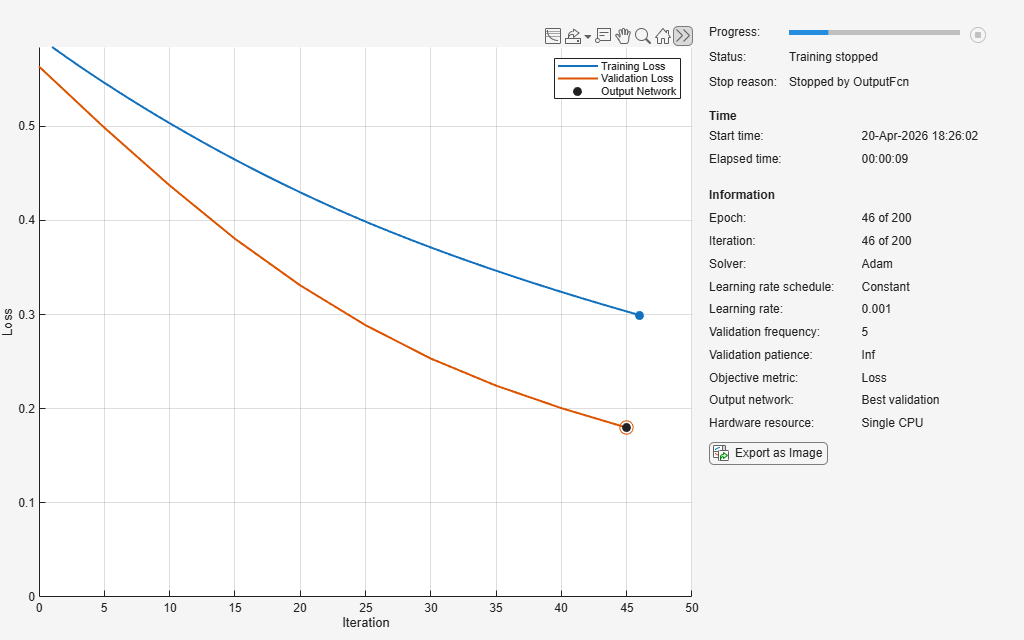# Custom Stopping Criteria for Deep Learning Training

Since R2023b

This example shows how to stop training of deep learning neural networks based on custom stopping criteria using `trainnet`.

You can specify neural network training options using `trainingOptions`. You can use validation data to stop training automatically when the validation loss stops decreasing. To turn on automatic validation stopping, use the `ValidationPatience` training option.

To stop training early when a custom criterion is met, pass a custom function handle to the `"OutputFcn"` name-value pair argument of `trainingOptions`. `trainnet` calls this function once before the start of training, after each training iteration, and once after training has finished. Each time the output functions are called, `trainnet` passes a structure containing information such as the current iteration number, loss, and accuracy. Training will stop when the custom output function returns `true`.

The network trained in this example classifies the gear tooth condition of a transmission system into two categories, `"Tooth Fault"` and `"No Tooth Fault"`, based on a mixture of numeric sensor readings, statistics, and categorical labels. For more information, see Train Neural Network with Tabular Data.

The custom output function defined in this example stops training early once the training loss is lower than the desired loss threshold.

### Load and Preprocess Training Data

Read the transmission casing data from the CSV file `"transmissionCasingData.csv"`.

```filename = "transmissionCasingData.csv"; tbl = readtable(filename,TextType="String");```

Convert the labels for prediction, and the categorical predictors to categorical using the `convertvars` function. In this data set, there are two categorical features, `"SensorCondition"` and `"ShaftCondition"`.

```labelName = "GearToothCondition"; categoricalPredictorNames = ["SensorCondition" "ShaftCondition"]; tbl = convertvars(tbl,[labelName categoricalPredictorNames],"categorical");```

To train a network using categorical features, you must convert the categorical features to numeric. You can do this using the `onehotencode` function.

```for i = 1:numel(categoricalPredictorNames) name = categoricalPredictorNames(i); tbl.(name) = onehotencode(tbl.(name),2); end```

Set aside data for testing. Partition the data into a training set containing 80% of the data, a validation set containing 10% of the data, and a test set containing the remaining 10% of the data. To partition the data, use the `trainingPartitions` function, attached to this example as a supporting file. To access this file, open the example as a live script.

```numObservations = size(tbl,1); [idxTrain,idxValidation,idxTest] = trainingPartitions(numObservations,[0.80 0.1 0.1]); tblTrain = tbl(idxTrain,:); tblValidation = tbl(idxValidation,:); tblTest = tbl(idxTest,:);```

Convert the data to a format that the `trainnet` function supports. Convert the predictors and targets to numeric and categorical arrays, respectively, using the `table2array`` `function.

```predictorNames = ["SigMean" "SigMedian" "SigRMS" "SigVar" "SigPeak" "SigPeak2Peak" ... "SigSkewness" "SigKurtosis" "SigCrestFactor" "SigMAD" "SigRangeCumSum" ... "SigCorrDimension" "SigApproxEntropy" "SigLyapExponent" "PeakFreq" ... "HighFreqPower" "EnvPower" "PeakSpecKurtosis" "SensorCondition" "ShaftCondition"]; XTrain = table2array(tblTrain(:,predictorNames)); TTrain = tblTrain.(labelName); XValidation = table2array(tblValidation(:,predictorNames)); TValidation = tblValidation.(labelName); XTest = table2array(tblTest(:,predictorNames)); TTest = tblTest.(labelName);```

### Network Architecture

Define the neural network architecture.

• For feature input, specify a feature input layer with the number of features. Normalize the input using Z-score normalization.

• Specify a fully connected layer with a size of 16, followed by a layer normalization and ReLU layer.

• For classification output, specify a fully connected layer with a size that matches the number of classes, followed by a softmax layer.

```numFeatures = size(XTrain,2); hiddenSize = 16; classNames = categories(tbl{:,labelName}); numClasses = numel(classNames); layers = [ featureInputLayer(numFeatures,Normalization="zscore") fullyConnectedLayer(hiddenSize) layerNormalizationLayer reluLayer fullyConnectedLayer(numClasses) softmaxLayer];```

### Define Training Options

Use the function `stopTraining` defined at the bottom of this page to stop training early when the training loss is smaller than a desired loss threshold. Use the `"OutputFcn"` name-value pair argument of `trainingOptions` to pass this function to `trainnet`.

Specify the training options:

• Train using the Adam solver.

• Train using the CPU. Because the network and data are small, the CPU is better suited.

• Validate the network every 5 iterations using the validation data.

• Set the maximum number of epochs to 200.

• Display the training progress in a plot.

• Suppress the verbose output.

• Include the custom output function `stopTraining`.

Define the loss threshold.

```lossThreshold = 0.3; options = trainingOptions("adam", ... ExecutionEnvironment="cpu", ... ValidationData={XValidation,TValidation}, ... ValidationFrequency=5, ... MaxEpochs=200, ... Plots="training-progress", ... Verbose=false, ... OutputFcn=@(info)stopTraining(info,lossThreshold));```

### Train Neural Network

Train the network.

`[net,info] = trainnet(XTrain,TTrain,layers,"crossentropy",options);`### Test Network

Predict the labels of the test data using the trained network. Predict the classification scores using the trained network then convert the predictions to labels using the `onehotdecode` function.

```scoresTest = predict(net,XTest); YTest = onehotdecode(scoresTest,classNames,2); accuracy = mean(YTest==TTest)```
```accuracy = 0.8636 ```

### Custom Output Function

Define the output function `stopTraining(info,lossThreshold)`, which stops training when the training loss is smaller than the loss threshold. Training stops when the output function returns `true`.

```function stop = stopTraining(info,lossThreshold) trainingLoss = info.TrainingLoss; stop = trainingLoss < lossThreshold; end```